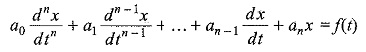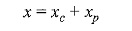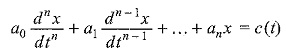## Non Homogeneous Differential Equation:

Now let us consider the following Non Homogeneous Differential Equation,where the coefficients a0, a1, … an are constants, and f(t) is a function of me.
The general solution may be writtenwhere xc is the complementary function, and xp is the particular integral. Since xc is the general solution of the corresponding homogeneous equation with f(t) replaced by zero, we have to find out the particular integral xp.

The particular integral can be calculated by the method of undetermined coefficients. This method is useful to equationswhen c(t) is such that the form of a particular solution xp of the above equation may be guessed.

For example, c(t) may be a single power of t, a polynomial, an exponential, a sinusoidal function, or a sum of such functions. The method consists in assuming for, xp an expression similar to that of c(t), containing unknown coefficients which are to be determined by inserting xp and its derivatives in the original equation.

Scroll to Top